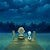# Exploratory Data Analysis (EDA) in Python

The goal of exploratory analysis is to find relationships between different variables in your data that you didn’t know that existed. You can do this by plotting your data and look for any trends, clusters or distributions. …

# Top Tips for Data Preparation Using Python

Hi friends , welcome to Top Tips for Data Preparation Using Python tutorial . so in this post we will learn an important topic of data science that is Data Preprocessing .

Pre-processing refers to the transformation applied to our data before feeding it to the algorithm .

Data Preprocessing…

# Introduction to Data Visualization in Python

Hi friends , welcome to Data Visualization python tutorial . so in this post we will learn an important topic of data science that is Data Visualization . Data visualization is the study to visualize data . so lets start how to visualize data in python.

# Data Visualization

• Data visualization is the…

# Classification Algorithm in Machine Learning

In machine learning and statistics , classification is a supervised learning approach in which the computer program learn form input data and then uses this learning to classify new observation .

Example : This chart shows the classification of the iris flower dataset into its three sub-species indicated by codes…

# Regression Techniques

Overview :

Learn about the different regression types in machine learning , including linear regression and logistic regression .

Each regression technique has its own regression equation and regression coefficients

What is regression Analysis ?

Regression analysis is a form of predictive modelling technique which investigates the relationship between a…

# Performing Analysis of Meteorological Data

Is there any change due in weather to global warming in of Finland by using Data analytics ?

My goal in this Internship (of data analysis) is to transform the raw data into information and then convert it into knowledge.

I am very excited to share with you my internship’s…